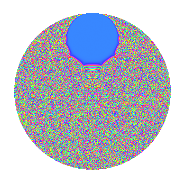# Properties

 Label 2.66.a.bLevel 2 Weight 66 Character orbit 2.a Self dual Yes Analytic conductor 53.514 Analytic rank 0 Dimension 3 CM No Inner twists 1

# Related objects

## Newspace parameters

 Level: $$N$$ = $$2$$ Weight: $$k$$ = $$66$$ Character orbit: $$[\chi]$$ = 2.a (trivial)

## Newform invariants

 Self dual: Yes Analytic conductor: $$53.5144712945$$ Analytic rank: $$0$$ Dimension: $$3$$ Coefficient field: $$\mathbb{Q}[x]/(x^{3} - \cdots)$$ Coefficient ring: $$\Z[a_1, \ldots, a_{5}]$$ Coefficient ring index: $$2^{22}\cdot 3^{8}\cdot 5^{3}\cdot 7\cdot 11\cdot 13$$ Fricke sign: $$-1$$ Sato-Tate group: $\mathrm{SU}(2)$

## $q$-expansion

Coefficients of the $$q$$-expansion are expressed in terms of a basis $$1,\beta_1,\beta_2$$ for the coefficient ring described below. We also show the integral $$q$$-expansion of the trace form.

 $$f(q)$$ $$=$$ $$q$$ $$+4294967296 q^{2}$$ $$+(994852866070404 - \beta_{1}) q^{3}$$ $$+18446744073709551616 q^{4}$$ $$+($$$$13\!\cdots\!50$$$$- 6038434 \beta_{1} - 13 \beta_{2}) q^{5}$$ $$+($$$$42\!\cdots\!84$$$$- 4294967296 \beta_{1}) q^{6}$$ $$+($$$$14\!\cdots\!88$$$$- 603582908362 \beta_{1} + 653660 \beta_{2}) q^{7}$$ $$+$$$$79\!\cdots\!36$$$$q^{8}$$ $$+($$$$28\!\cdots\!73$$$$- 3475318834685628 \beta_{1} + 1845485370 \beta_{2}) q^{9}$$ $$+O(q^{10})$$ $$q$$ $$+4294967296 q^{2}$$ $$+(994852866070404 - \beta_{1}) q^{3}$$ $$+18446744073709551616 q^{4}$$ $$+($$$$13\!\cdots\!50$$$$- 6038434 \beta_{1} - 13 \beta_{2}) q^{5}$$ $$+($$$$42\!\cdots\!84$$$$- 4294967296 \beta_{1}) q^{6}$$ $$+($$$$14\!\cdots\!88$$$$- 603582908362 \beta_{1} + 653660 \beta_{2}) q^{7}$$ $$+$$$$79\!\cdots\!36$$$$q^{8}$$ $$+($$$$28\!\cdots\!73$$$$- 3475318834685628 \beta_{1} + 1845485370 \beta_{2}) q^{9}$$ $$+($$$$56\!\cdots\!00$$$$- 25934876549054464 \beta_{1} - 55834574848 \beta_{2}) q^{10}$$ $$+(-$$$$65\!\cdots\!28$$$$- 73952434140039763 \beta_{1} - 1635827959720 \beta_{2}) q^{11}$$ $$+($$$$18\!\cdots\!64$$$$- 18446744073709551616 \beta_{1}) q^{12}$$ $$+($$$$19\!\cdots\!14$$$$+$$$$23\!\cdots\!58$$$$\beta_{1} - 347185116853685 \beta_{2}) q^{13}$$ $$+($$$$61\!\cdots\!48$$$$-$$$$25\!\cdots\!52$$$$\beta_{1} + 2807448322703360 \beta_{2}) q^{14}$$ $$+($$$$74\!\cdots\!00$$$$+$$$$10\!\cdots\!34$$$$\beta_{1} + 17523724678877988 \beta_{2}) q^{15}$$ $$+$$$$34\!\cdots\!56$$$$q^{16}$$ $$+(-$$$$15\!\cdots\!82$$$$-$$$$16\!\cdots\!08$$$$\beta_{1} - 2224277365667729270 \beta_{2}) q^{17}$$ $$+($$$$12\!\cdots\!08$$$$-$$$$14\!\cdots\!88$$$$\beta_{1} + 7926299309396459520 \beta_{2}) q^{18}$$ $$+(-$$$$25\!\cdots\!60$$$$-$$$$51\!\cdots\!93$$$$\beta_{1} + 37348845711370692520 \beta_{2}) q^{19}$$ $$+($$$$24\!\cdots\!00$$$$-$$$$11\!\cdots\!44$$$$\beta_{1} -$$$$23\!\cdots\!08$$$$\beta_{2}) q^{20}$$ $$+($$$$87\!\cdots\!52$$$$-$$$$42\!\cdots\!76$$$$\beta_{1} +$$$$79\!\cdots\!80$$$$\beta_{2}) q^{21}$$ $$+(-$$$$28\!\cdots\!88$$$$-$$$$31\!\cdots\!48$$$$\beta_{1} -$$$$70\!\cdots\!20$$$$\beta_{2}) q^{22}$$ $$+(-$$$$82\!\cdots\!56$$$$+$$$$67\!\cdots\!74$$$$\beta_{1} +$$$$30\!\cdots\!60$$$$\beta_{2}) q^{23}$$ $$+($$$$78\!\cdots\!44$$$$-$$$$79\!\cdots\!36$$$$\beta_{1}) q^{24}$$ $$+($$$$64\!\cdots\!75$$$$+$$$$54\!\cdots\!00$$$$\beta_{1} -$$$$87\!\cdots\!00$$$$\beta_{2}) q^{25}$$ $$+($$$$85\!\cdots\!44$$$$+$$$$10\!\cdots\!68$$$$\beta_{1} -$$$$14\!\cdots\!60$$$$\beta_{2}) q^{26}$$ $$+($$$$34\!\cdots\!20$$$$-$$$$50\!\cdots\!02$$$$\beta_{1} +$$$$55\!\cdots\!40$$$$\beta_{2}) q^{27}$$ $$+($$$$26\!\cdots\!08$$$$-$$$$11\!\cdots\!92$$$$\beta_{1} +$$$$12\!\cdots\!60$$$$\beta_{2}) q^{28}$$ $$+($$$$17\!\cdots\!70$$$$-$$$$60\!\cdots\!86$$$$\beta_{1} -$$$$84\!\cdots\!05$$$$\beta_{2}) q^{29}$$ $$+($$$$32\!\cdots\!00$$$$+$$$$47\!\cdots\!64$$$$\beta_{1} +$$$$75\!\cdots\!48$$$$\beta_{2}) q^{30}$$ $$+($$$$20\!\cdots\!92$$$$+$$$$62\!\cdots\!04$$$$\beta_{1} +$$$$15\!\cdots\!80$$$$\beta_{2}) q^{31}$$ $$+$$$$14\!\cdots\!76$$$$q^{32}$$ $$+($$$$83\!\cdots\!88$$$$+$$$$33\!\cdots\!16$$$$\beta_{1} +$$$$93\!\cdots\!30$$$$\beta_{2}) q^{33}$$ $$+(-$$$$65\!\cdots\!72$$$$-$$$$72\!\cdots\!68$$$$\beta_{1} -$$$$95\!\cdots\!20$$$$\beta_{2}) q^{34}$$ $$+(-$$$$71\!\cdots\!00$$$$-$$$$21\!\cdots\!52$$$$\beta_{1} +$$$$12\!\cdots\!36$$$$\beta_{2}) q^{35}$$ $$+($$$$52\!\cdots\!68$$$$-$$$$64\!\cdots\!48$$$$\beta_{1} +$$$$34\!\cdots\!20$$$$\beta_{2}) q^{36}$$ $$+(-$$$$54\!\cdots\!02$$$$+$$$$23\!\cdots\!02$$$$\beta_{1} -$$$$99\!\cdots\!45$$$$\beta_{2}) q^{37}$$ $$+(-$$$$10\!\cdots\!60$$$$-$$$$21\!\cdots\!28$$$$\beta_{1} +$$$$16\!\cdots\!20$$$$\beta_{2}) q^{38}$$ $$+(-$$$$26\!\cdots\!44$$$$+$$$$11\!\cdots\!78$$$$\beta_{1} -$$$$26\!\cdots\!00$$$$\beta_{2}) q^{39}$$ $$+($$$$10\!\cdots\!00$$$$-$$$$47\!\cdots\!24$$$$\beta_{1} -$$$$10\!\cdots\!68$$$$\beta_{2}) q^{40}$$ $$+($$$$12\!\cdots\!62$$$$+$$$$14\!\cdots\!16$$$$\beta_{1} +$$$$40\!\cdots\!60$$$$\beta_{2}) q^{41}$$ $$+($$$$37\!\cdots\!92$$$$-$$$$18\!\cdots\!96$$$$\beta_{1} +$$$$34\!\cdots\!80$$$$\beta_{2}) q^{42}$$ $$+($$$$66\!\cdots\!64$$$$+$$$$12\!\cdots\!97$$$$\beta_{1} -$$$$18\!\cdots\!40$$$$\beta_{2}) q^{43}$$ $$+(-$$$$12\!\cdots\!48$$$$-$$$$13\!\cdots\!08$$$$\beta_{1} -$$$$30\!\cdots\!20$$$$\beta_{2}) q^{44}$$ $$+(-$$$$72\!\cdots\!50$$$$-$$$$21\!\cdots\!22$$$$\beta_{1} +$$$$10\!\cdots\!71$$$$\beta_{2}) q^{45}$$ $$+(-$$$$35\!\cdots\!76$$$$+$$$$28\!\cdots\!04$$$$\beta_{1} +$$$$12\!\cdots\!60$$$$\beta_{2}) q^{46}$$ $$+(-$$$$51\!\cdots\!52$$$$+$$$$13\!\cdots\!08$$$$\beta_{1} -$$$$48\!\cdots\!80$$$$\beta_{2}) q^{47}$$ $$+($$$$33\!\cdots\!24$$$$-$$$$34\!\cdots\!56$$$$\beta_{1}) q^{48}$$ $$+($$$$38\!\cdots\!37$$$$-$$$$32\!\cdots\!92$$$$\beta_{1} +$$$$47\!\cdots\!40$$$$\beta_{2}) q^{49}$$ $$+($$$$27\!\cdots\!00$$$$+$$$$23\!\cdots\!00$$$$\beta_{1} -$$$$37\!\cdots\!00$$$$\beta_{2}) q^{50}$$ $$+($$$$19\!\cdots\!72$$$$+$$$$19\!\cdots\!90$$$$\beta_{1} +$$$$42\!\cdots\!80$$$$\beta_{2}) q^{51}$$ $$+($$$$36\!\cdots\!24$$$$+$$$$43\!\cdots\!28$$$$\beta_{1} -$$$$64\!\cdots\!60$$$$\beta_{2}) q^{52}$$ $$+($$$$12\!\cdots\!94$$$$+$$$$60\!\cdots\!18$$$$\beta_{1} -$$$$92\!\cdots\!45$$$$\beta_{2}) q^{53}$$ $$+($$$$14\!\cdots\!20$$$$-$$$$21\!\cdots\!92$$$$\beta_{1} +$$$$23\!\cdots\!40$$$$\beta_{2}) q^{54}$$ $$+($$$$29\!\cdots\!00$$$$+$$$$58\!\cdots\!62$$$$\beta_{1} -$$$$28\!\cdots\!16$$$$\beta_{2}) q^{55}$$ $$+($$$$11\!\cdots\!68$$$$-$$$$47\!\cdots\!32$$$$\beta_{1} +$$$$51\!\cdots\!60$$$$\beta_{2}) q^{56}$$ $$+($$$$59\!\cdots\!60$$$$-$$$$17\!\cdots\!72$$$$\beta_{1} +$$$$76\!\cdots\!90$$$$\beta_{2}) q^{57}$$ $$+($$$$76\!\cdots\!20$$$$-$$$$26\!\cdots\!56$$$$\beta_{1} -$$$$36\!\cdots\!80$$$$\beta_{2}) q^{58}$$ $$+($$$$33\!\cdots\!40$$$$+$$$$56\!\cdots\!73$$$$\beta_{1} +$$$$15\!\cdots\!40$$$$\beta_{2}) q^{59}$$ $$+($$$$13\!\cdots\!00$$$$+$$$$20\!\cdots\!44$$$$\beta_{1} +$$$$32\!\cdots\!08$$$$\beta_{2}) q^{60}$$ $$+($$$$10\!\cdots\!82$$$$+$$$$21\!\cdots\!38$$$$\beta_{1} -$$$$33\!\cdots\!65$$$$\beta_{2}) q^{61}$$ $$+($$$$86\!\cdots\!32$$$$+$$$$26\!\cdots\!84$$$$\beta_{1} +$$$$67\!\cdots\!80$$$$\beta_{2}) q^{62}$$ $$+($$$$46\!\cdots\!24$$$$-$$$$14\!\cdots\!10$$$$\beta_{1} +$$$$80\!\cdots\!60$$$$\beta_{2}) q^{63}$$ $$+$$$$62\!\cdots\!96$$$$q^{64}$$ $$+($$$$45\!\cdots\!00$$$$+$$$$67\!\cdots\!64$$$$\beta_{1} -$$$$16\!\cdots\!52$$$$\beta_{2}) q^{65}$$ $$+($$$$35\!\cdots\!48$$$$+$$$$14\!\cdots\!36$$$$\beta_{1} +$$$$40\!\cdots\!80$$$$\beta_{2}) q^{66}$$ $$+($$$$57\!\cdots\!88$$$$-$$$$95\!\cdots\!61$$$$\beta_{1} +$$$$75\!\cdots\!20$$$$\beta_{2}) q^{67}$$ $$+(-$$$$27\!\cdots\!12$$$$-$$$$31\!\cdots\!28$$$$\beta_{1} -$$$$41\!\cdots\!20$$$$\beta_{2}) q^{68}$$ $$+(-$$$$89\!\cdots\!24$$$$+$$$$18\!\cdots\!32$$$$\beta_{1} -$$$$13\!\cdots\!40$$$$\beta_{2}) q^{69}$$ $$+(-$$$$30\!\cdots\!00$$$$-$$$$90\!\cdots\!92$$$$\beta_{1} +$$$$54\!\cdots\!56$$$$\beta_{2}) q^{70}$$ $$+(-$$$$68\!\cdots\!68$$$$-$$$$10\!\cdots\!82$$$$\beta_{1} +$$$$86\!\cdots\!80$$$$\beta_{2}) q^{71}$$ $$+($$$$22\!\cdots\!28$$$$-$$$$27\!\cdots\!08$$$$\beta_{1} +$$$$14\!\cdots\!20$$$$\beta_{2}) q^{72}$$ $$+(-$$$$49\!\cdots\!66$$$$-$$$$64\!\cdots\!72$$$$\beta_{1} +$$$$17\!\cdots\!30$$$$\beta_{2}) q^{73}$$ $$+(-$$$$23\!\cdots\!92$$$$+$$$$99\!\cdots\!92$$$$\beta_{1} -$$$$42\!\cdots\!20$$$$\beta_{2}) q^{74}$$ $$+(-$$$$65\!\cdots\!00$$$$+$$$$14\!\cdots\!25$$$$\beta_{1} -$$$$96\!\cdots\!00$$$$\beta_{2}) q^{75}$$ $$+(-$$$$47\!\cdots\!60$$$$-$$$$94\!\cdots\!88$$$$\beta_{1} +$$$$68\!\cdots\!20$$$$\beta_{2}) q^{76}$$ $$+(-$$$$14\!\cdots\!64$$$$+$$$$31\!\cdots\!72$$$$\beta_{1} +$$$$13\!\cdots\!80$$$$\beta_{2}) q^{77}$$ $$+(-$$$$11\!\cdots\!24$$$$+$$$$48\!\cdots\!88$$$$\beta_{1} -$$$$11\!\cdots\!00$$$$\beta_{2}) q^{78}$$ $$+($$$$20\!\cdots\!00$$$$-$$$$70\!\cdots\!28$$$$\beta_{1} +$$$$47\!\cdots\!40$$$$\beta_{2}) q^{79}$$ $$+($$$$44\!\cdots\!00$$$$-$$$$20\!\cdots\!04$$$$\beta_{1} -$$$$44\!\cdots\!28$$$$\beta_{2}) q^{80}$$ $$+($$$$66\!\cdots\!41$$$$-$$$$22\!\cdots\!64$$$$\beta_{1} -$$$$12\!\cdots\!10$$$$\beta_{2}) q^{81}$$ $$+($$$$52\!\cdots\!52$$$$+$$$$62\!\cdots\!36$$$$\beta_{1} +$$$$17\!\cdots\!60$$$$\beta_{2}) q^{82}$$ $$+($$$$68\!\cdots\!24$$$$+$$$$70\!\cdots\!15$$$$\beta_{1} -$$$$12\!\cdots\!20$$$$\beta_{2}) q^{83}$$ $$+($$$$16\!\cdots\!32$$$$-$$$$79\!\cdots\!16$$$$\beta_{1} +$$$$14\!\cdots\!80$$$$\beta_{2}) q^{84}$$ $$+($$$$52\!\cdots\!00$$$$+$$$$11\!\cdots\!48$$$$\beta_{1} +$$$$27\!\cdots\!86$$$$\beta_{2}) q^{85}$$ $$+($$$$28\!\cdots\!44$$$$+$$$$52\!\cdots\!12$$$$\beta_{1} -$$$$81\!\cdots\!40$$$$\beta_{2}) q^{86}$$ $$+($$$$91\!\cdots\!80$$$$-$$$$15\!\cdots\!34$$$$\beta_{1} +$$$$15\!\cdots\!00$$$$\beta_{2}) q^{87}$$ $$+(-$$$$51\!\cdots\!08$$$$-$$$$58\!\cdots\!68$$$$\beta_{1} -$$$$12\!\cdots\!20$$$$\beta_{2}) q^{88}$$ $$+(-$$$$37\!\cdots\!10$$$$-$$$$56\!\cdots\!40$$$$\beta_{1} -$$$$39\!\cdots\!50$$$$\beta_{2}) q^{89}$$ $$+(-$$$$31\!\cdots\!00$$$$-$$$$94\!\cdots\!12$$$$\beta_{1} +$$$$45\!\cdots\!16$$$$\beta_{2}) q^{90}$$ $$+(-$$$$45\!\cdots\!68$$$$+$$$$81\!\cdots\!56$$$$\beta_{1} +$$$$38\!\cdots\!40$$$$\beta_{2}) q^{91}$$ $$+(-$$$$15\!\cdots\!96$$$$+$$$$12\!\cdots\!84$$$$\beta_{1} +$$$$55\!\cdots\!60$$$$\beta_{2}) q^{92}$$ $$+(-$$$$55\!\cdots\!32$$$$-$$$$79\!\cdots\!96$$$$\beta_{1} -$$$$12\!\cdots\!60$$$$\beta_{2}) q^{93}$$ $$+(-$$$$22\!\cdots\!92$$$$+$$$$56\!\cdots\!68$$$$\beta_{1} -$$$$20\!\cdots\!80$$$$\beta_{2}) q^{94}$$ $$+(-$$$$29\!\cdots\!00$$$$-$$$$26\!\cdots\!50$$$$\beta_{1} +$$$$26\!\cdots\!00$$$$\beta_{2}) q^{95}$$ $$+($$$$14\!\cdots\!04$$$$-$$$$14\!\cdots\!76$$$$\beta_{1}) q^{96}$$ $$+(-$$$$24\!\cdots\!82$$$$+$$$$12\!\cdots\!00$$$$\beta_{1} -$$$$11\!\cdots\!30$$$$\beta_{2}) q^{97}$$ $$+($$$$16\!\cdots\!52$$$$-$$$$13\!\cdots\!32$$$$\beta_{1} +$$$$20\!\cdots\!40$$$$\beta_{2}) q^{98}$$ $$+(-$$$$38\!\cdots\!44$$$$+$$$$61\!\cdots\!05$$$$\beta_{1} +$$$$10\!\cdots\!60$$$$\beta_{2}) q^{99}$$ $$+O(q^{100})$$ $$\operatorname{Tr}(f)(q)$$ $$=$$ $$3q$$ $$\mathstrut +\mathstrut 12884901888q^{2}$$ $$\mathstrut +\mathstrut 2984558598211212q^{3}$$ $$\mathstrut +\mathstrut 55340232221128654848q^{4}$$ $$\mathstrut +\mathstrut$$$$39\!\cdots\!50$$$$q^{5}$$ $$\mathstrut +\mathstrut$$$$12\!\cdots\!52$$$$q^{6}$$ $$\mathstrut +\mathstrut$$$$42\!\cdots\!64$$$$q^{7}$$ $$\mathstrut +\mathstrut$$$$23\!\cdots\!08$$$$q^{8}$$ $$\mathstrut +\mathstrut$$$$85\!\cdots\!19$$$$q^{9}$$ $$\mathstrut +\mathstrut O(q^{10})$$ $$3q$$ $$\mathstrut +\mathstrut 12884901888q^{2}$$ $$\mathstrut +\mathstrut 2984558598211212q^{3}$$ $$\mathstrut +\mathstrut 55340232221128654848q^{4}$$ $$\mathstrut +\mathstrut$$$$39\!\cdots\!50$$$$q^{5}$$ $$\mathstrut +\mathstrut$$$$12\!\cdots\!52$$$$q^{6}$$ $$\mathstrut +\mathstrut$$$$42\!\cdots\!64$$$$q^{7}$$ $$\mathstrut +\mathstrut$$$$23\!\cdots\!08$$$$q^{8}$$ $$\mathstrut +\mathstrut$$$$85\!\cdots\!19$$$$q^{9}$$ $$\mathstrut +\mathstrut$$$$16\!\cdots\!00$$$$q^{10}$$ $$\mathstrut -\mathstrut$$$$19\!\cdots\!84$$$$q^{11}$$ $$\mathstrut +\mathstrut$$$$55\!\cdots\!92$$$$q^{12}$$ $$\mathstrut +\mathstrut$$$$59\!\cdots\!42$$$$q^{13}$$ $$\mathstrut +\mathstrut$$$$18\!\cdots\!44$$$$q^{14}$$ $$\mathstrut +\mathstrut$$$$22\!\cdots\!00$$$$q^{15}$$ $$\mathstrut +\mathstrut$$$$10\!\cdots\!68$$$$q^{16}$$ $$\mathstrut -\mathstrut$$$$45\!\cdots\!46$$$$q^{17}$$ $$\mathstrut +\mathstrut$$$$36\!\cdots\!24$$$$q^{18}$$ $$\mathstrut -\mathstrut$$$$76\!\cdots\!80$$$$q^{19}$$ $$\mathstrut +\mathstrut$$$$72\!\cdots\!00$$$$q^{20}$$ $$\mathstrut +\mathstrut$$$$26\!\cdots\!56$$$$q^{21}$$ $$\mathstrut -\mathstrut$$$$84\!\cdots\!64$$$$q^{22}$$ $$\mathstrut -\mathstrut$$$$24\!\cdots\!68$$$$q^{23}$$ $$\mathstrut +\mathstrut$$$$23\!\cdots\!32$$$$q^{24}$$ $$\mathstrut +\mathstrut$$$$19\!\cdots\!25$$$$q^{25}$$ $$\mathstrut +\mathstrut$$$$25\!\cdots\!32$$$$q^{26}$$ $$\mathstrut +\mathstrut$$$$10\!\cdots\!60$$$$q^{27}$$ $$\mathstrut +\mathstrut$$$$79\!\cdots\!24$$$$q^{28}$$ $$\mathstrut +\mathstrut$$$$53\!\cdots\!10$$$$q^{29}$$ $$\mathstrut +\mathstrut$$$$96\!\cdots\!00$$$$q^{30}$$ $$\mathstrut +\mathstrut$$$$60\!\cdots\!76$$$$q^{31}$$ $$\mathstrut +\mathstrut$$$$43\!\cdots\!28$$$$q^{32}$$ $$\mathstrut +\mathstrut$$$$24\!\cdots\!64$$$$q^{33}$$ $$\mathstrut -\mathstrut$$$$19\!\cdots\!16$$$$q^{34}$$ $$\mathstrut -\mathstrut$$$$21\!\cdots\!00$$$$q^{35}$$ $$\mathstrut +\mathstrut$$$$15\!\cdots\!04$$$$q^{36}$$ $$\mathstrut -\mathstrut$$$$16\!\cdots\!06$$$$q^{37}$$ $$\mathstrut -\mathstrut$$$$32\!\cdots\!80$$$$q^{38}$$ $$\mathstrut -\mathstrut$$$$80\!\cdots\!32$$$$q^{39}$$ $$\mathstrut +\mathstrut$$$$31\!\cdots\!00$$$$q^{40}$$ $$\mathstrut +\mathstrut$$$$36\!\cdots\!86$$$$q^{41}$$ $$\mathstrut +\mathstrut$$$$11\!\cdots\!76$$$$q^{42}$$ $$\mathstrut +\mathstrut$$$$19\!\cdots\!92$$$$q^{43}$$ $$\mathstrut -\mathstrut$$$$36\!\cdots\!44$$$$q^{44}$$ $$\mathstrut -\mathstrut$$$$21\!\cdots\!50$$$$q^{45}$$ $$\mathstrut -\mathstrut$$$$10\!\cdots\!28$$$$q^{46}$$ $$\mathstrut -\mathstrut$$$$15\!\cdots\!56$$$$q^{47}$$ $$\mathstrut +\mathstrut$$$$10\!\cdots\!72$$$$q^{48}$$ $$\mathstrut +\mathstrut$$$$11\!\cdots\!11$$$$q^{49}$$ $$\mathstrut +\mathstrut$$$$83\!\cdots\!00$$$$q^{50}$$ $$\mathstrut +\mathstrut$$$$57\!\cdots\!16$$$$q^{51}$$ $$\mathstrut +\mathstrut$$$$10\!\cdots\!72$$$$q^{52}$$ $$\mathstrut +\mathstrut$$$$38\!\cdots\!82$$$$q^{53}$$ $$\mathstrut +\mathstrut$$$$44\!\cdots\!60$$$$q^{54}$$ $$\mathstrut +\mathstrut$$$$89\!\cdots\!00$$$$q^{55}$$ $$\mathstrut +\mathstrut$$$$34\!\cdots\!04$$$$q^{56}$$ $$\mathstrut +\mathstrut$$$$17\!\cdots\!80$$$$q^{57}$$ $$\mathstrut +\mathstrut$$$$22\!\cdots\!60$$$$q^{58}$$ $$\mathstrut +\mathstrut$$$$99\!\cdots\!20$$$$q^{59}$$ $$\mathstrut +\mathstrut$$$$41\!\cdots\!00$$$$q^{60}$$ $$\mathstrut +\mathstrut$$$$30\!\cdots\!46$$$$q^{61}$$ $$\mathstrut +\mathstrut$$$$25\!\cdots\!96$$$$q^{62}$$ $$\mathstrut +\mathstrut$$$$13\!\cdots\!72$$$$q^{63}$$ $$\mathstrut +\mathstrut$$$$18\!\cdots\!88$$$$q^{64}$$ $$\mathstrut +\mathstrut$$$$13\!\cdots\!00$$$$q^{65}$$ $$\mathstrut +\mathstrut$$$$10\!\cdots\!44$$$$q^{66}$$ $$\mathstrut +\mathstrut$$$$17\!\cdots\!64$$$$q^{67}$$ $$\mathstrut -\mathstrut$$$$83\!\cdots\!36$$$$q^{68}$$ $$\mathstrut -\mathstrut$$$$26\!\cdots\!72$$$$q^{69}$$ $$\mathstrut -\mathstrut$$$$91\!\cdots\!00$$$$q^{70}$$ $$\mathstrut -\mathstrut$$$$20\!\cdots\!04$$$$q^{71}$$ $$\mathstrut +\mathstrut$$$$67\!\cdots\!84$$$$q^{72}$$ $$\mathstrut -\mathstrut$$$$14\!\cdots\!98$$$$q^{73}$$ $$\mathstrut -\mathstrut$$$$69\!\cdots\!76$$$$q^{74}$$ $$\mathstrut -\mathstrut$$$$19\!\cdots\!00$$$$q^{75}$$ $$\mathstrut -\mathstrut$$$$14\!\cdots\!80$$$$q^{76}$$ $$\mathstrut -\mathstrut$$$$42\!\cdots\!92$$$$q^{77}$$ $$\mathstrut -\mathstrut$$$$34\!\cdots\!72$$$$q^{78}$$ $$\mathstrut +\mathstrut$$$$61\!\cdots\!00$$$$q^{79}$$ $$\mathstrut +\mathstrut$$$$13\!\cdots\!00$$$$q^{80}$$ $$\mathstrut +\mathstrut$$$$19\!\cdots\!23$$$$q^{81}$$ $$\mathstrut +\mathstrut$$$$15\!\cdots\!56$$$$q^{82}$$ $$\mathstrut +\mathstrut$$$$20\!\cdots\!72$$$$q^{83}$$ $$\mathstrut +\mathstrut$$$$48\!\cdots\!96$$$$q^{84}$$ $$\mathstrut +\mathstrut$$$$15\!\cdots\!00$$$$q^{85}$$ $$\mathstrut +\mathstrut$$$$85\!\cdots\!32$$$$q^{86}$$ $$\mathstrut +\mathstrut$$$$27\!\cdots\!40$$$$q^{87}$$ $$\mathstrut -\mathstrut$$$$15\!\cdots\!24$$$$q^{88}$$ $$\mathstrut -\mathstrut$$$$11\!\cdots\!30$$$$q^{89}$$ $$\mathstrut -\mathstrut$$$$93\!\cdots\!00$$$$q^{90}$$ $$\mathstrut -\mathstrut$$$$13\!\cdots\!04$$$$q^{91}$$ $$\mathstrut -\mathstrut$$$$45\!\cdots\!88$$$$q^{92}$$ $$\mathstrut -\mathstrut$$$$16\!\cdots\!96$$$$q^{93}$$ $$\mathstrut -\mathstrut$$$$66\!\cdots\!76$$$$q^{94}$$ $$\mathstrut -\mathstrut$$$$89\!\cdots\!00$$$$q^{95}$$ $$\mathstrut +\mathstrut$$$$43\!\cdots\!12$$$$q^{96}$$ $$\mathstrut -\mathstrut$$$$74\!\cdots\!46$$$$q^{97}$$ $$\mathstrut +\mathstrut$$$$49\!\cdots\!56$$$$q^{98}$$ $$\mathstrut -\mathstrut$$$$11\!\cdots\!32$$$$q^{99}$$ $$\mathstrut +\mathstrut O(q^{100})$$

Basis of coefficient ring in terms of a root $$\nu$$ of $$x^{3}\mathstrut -\mathstrut$$ $$x^{2}\mathstrut -\mathstrut$$ $$4862367805520722608042$$ $$x\mathstrut +\mathstrut$$ $$130125819203569060903952569933488$$:

 $$\beta_{0}$$ $$=$$ $$1$$ $$\beta_{1}$$ $$=$$ $$($$$$8640 \nu^{2} + 341742997270080 \nu - 28007238559913276554748160$$$$)/$$$$439529881$$ $$\beta_{2}$$ $$=$$ $$($$$$253376640 \nu^{2} + 14192563459783927680 \nu - 821340278009406951018491727360$$$$)/62789983$$
 $$1$$ $$=$$ $$\beta_0$$ $$\nu$$ $$=$$ $$($$$$\beta_{2}\mathstrut -\mathstrut$$ $$205282$$ $$\beta_{1}\mathstrut +\mathstrut$$ $$22140518400$$$$)/$$$$66421555200$$ $$\nu^{2}$$ $$=$$ $$($$$$-$$$$5650512521$$ $$\beta_{2}\mathstrut +\mathstrut$$ $$1642657807845362$$ $$\beta_{1}\mathstrut +\mathstrut$$ $$30758669675914051567045988352000$$$$)/$$$$9488793600$$

## Embeddings

For each embedding $$\iota_m$$ of the coefficient field, the values $$\iota_m(a_n)$$ are shown below.

For more information on an embedded modular form you can click on its label.

Label $$\iota_m(\nu)$$ $$a_{2}$$ $$a_{3}$$ $$a_{4}$$ $$a_{5}$$ $$a_{6}$$ $$a_{7}$$ $$a_{8}$$ $$a_{9}$$ $$a_{10}$$
1.1
 4.20131e10 −8.04922e10 3.84791e10
4.29497e9 −2.64736e15 1.84467e19 −6.66845e22 −1.13703e25 1.54537e27 7.92282e28 −3.29254e30 −2.86408e32
1.2 4.29497e9 −5.99699e13 1.84467e19 6.16250e22 −2.57569e23 −2.55894e27 7.92282e28 −1.02975e31 2.64677e32
1.3 4.29497e9 5.69189e15 1.84467e19 8.97760e21 2.44465e25 5.30637e27 7.92282e28 2.20965e31 3.85585e31
 $$n$$: e.g. 2-40 or 990-1000 Significant digits: Format: Complex embeddings Normalized embeddings Satake parameters Satake angles

## Inner twists

This newform does not admit any (nontrivial) inner twists.

## Atkin-Lehner signs

$$p$$ Sign
$$2$$ $$-1$$

## Hecke kernels

This newform can be constructed as the kernel of the linear operator $$T_{3}^{3}$$ $$\mathstrut -\mathstrut$$$$29\!\cdots\!12$$$$T_{3}^{2}$$ $$\mathstrut -\mathstrut$$$$15\!\cdots\!52$$$$T_{3}$$ $$\mathstrut -\mathstrut$$$$90\!\cdots\!64$$ acting on $$S_{66}^{\mathrm{new}}(\Gamma_0(2))$$.